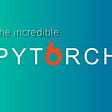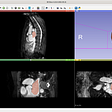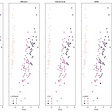# K-Means Clustering: Simple intuition

A simple and intuitive approach to understand K-Means algorithm.

What is Clustering?
Clustering is a sort of a task where each data point is clubbed with “Similar” data points and they form one cluster. In other words, data points in one cluster or group are very “Similar” to each other compared to the data points present in another cluster.

K-Means clustering is a very powerful and effective unsupervised machine learning algorithm.

Simple intuition of K-Means Clustering

K-means intends to divide ’n’ number of points into ‘K’ clusters where each point in cluster ‘Xi’ is similar or we can say have small ‘intra-cluster distance’ and have high ‘inter-cluster distance’. This is the basic intuition of K-means clustering.
Intra-cluster distance:- points which are in the same group or cluster.
Inter-cluster distance:- points across different clusters.

The ideal cluster would be a cluster having very small intra-cluster distance and high inter-cluster distance.

K-means groups points into the cluster. Now for each cluster it gives centroids C1,C2,…….,Ck where k=number of clusters. Centroid is nothing but the mean point(central point).Hence for each cluster we get one centroid and also the intersection of two different clusters is always a null set.
Here the number of clusters is the hyperparameter.

As we can see in the figure above, the pinkish point in every cluster is the centroid and points near to that centroid are grouped together.

Mathematical formulation of K-Means

The above formulation says find me the centroid, for all the clusters and for all the points belong to some set Sj where the intra-cluster distance is minimized such that data point x(which is Xi mentioned in the blog, please don't get confused)should belong to that set Sj only and intersection of any two sets should be a null set.

It is aright if you get little confused with the formulation, just keep in mind what we are trying to achieve and what the task is.

The task is how to find the K-centroids. We will use Lloyd’s algorithm to determine.

Algorithm:-

Initialization: We first randomly pick K points from our dataset and call them centroids. This is also called as Random Initialization Scheme.

Assignment: For each point ‘Xi’:- select the nearest centroid(according to the Euclidean distance function) and create a set.

Update Stage: Recalculate or update centroid by taking the mean of all the points in the particular set and make that new centroid.

Convergence stage: Repeat the Assignment and Update Stage until convergence.

What is convergence here? It is when the centroids, when updated, don't change much. Basically the centroids in previous stage and the next stage are the same or roughly same then that is the convergence stage.

At the end we have the centroids and the set of points in a cluster.

I am pretty sure with the following example one can easily understand how K-means actually work.

Let assume we want to group shopping habits just based on the ages of people. We are using one-dimensional dataset for simplicity.
Ages: 20,22,24,28,30,34,55,58,61,66.

Here n=10 and we want 2 clusters, so K=2.

We randomly take 2 centroids as we want two clusters. Lets say we took c1=21 and c2=30

Distance 1= | xi-c1 |
Distance 2= | xi-c2 |

After 1st iteration:

Our new centroids would be c1=22 and c2=47.42
The new centroids are calculated by taking mean of all the x present in cluster 1 and 2 respectively.

After 2nd iteration.

Our new centroids would be c1=26.33 and c2=60
The new centroids are calculated by taking mean of all the x present in cluster 1 and 2 respectively.

After 3rd iteration:

As we can see the new centroids in the 2nd iteration and the 3rd iteration is the same so we have reached the convergence stage or the convergence point.

So we have identified one group age 20–34 and second group stage 55–66.

This is just a toy dataset and it not need to be this simple in real life. This is taken into consideration for better understanding on how K-Means clustering work.

I hope you got a better intuition on how K-Means clustering work.

If you love this blog then do clap and feel free to drop any query or any feedback. Would be happy to help.

Also do follow for more blogs and insightful stories.

Reference:
AppliedAI course for giving a simple and clear intuition behind K-means.

Thank you.

--

--

--

## More from Analytics Vidhya

Analytics Vidhya is a community of Analytics and Data Science professionals. We are building the next-gen data science ecosystem https://www.analyticsvidhya.com

## Starter Pack for Deep Learning in PyTorch — for Extreme Beginners -by a beginner!## Backpropagation and Vanishing gradient problem in RNN clearly explained## Text-Driven Image Manipulation/Generation with CLIP## An application of Numerical Solutions to Maximum Likelihood Estimation in GraphSLAM## MONAI v0.6 and MONAI Label v0.1## EXTRACTING INFORMATION FROM MEDICINE USING DEEP LEARNING AND COMPUTER VISION## Should You Buy MacBook M1 for Machine Learning?## BERT + NLP: Turning Toward Innovation Research## Vihaanshah

A novice data scientist trying to 'script' my way into the programming world.

## Holiday Package Prediction## 4 Clustering Model Algorithms in Python and Which is the Best## SMS spam classification using Naïve Bayes Classifier## Evaluation of Classification Model